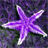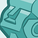# QlikView App Dev

Discussion Board for collaboration related to QlikView App Development.

Announcements
Action-Packed Learning Awaits! QlikWorld 2023. April 17 - 20 in Las Vegas: REGISTER NOW
cancel
Showing results for
Did you mean:Creator

## Forecasting in Qlikview

Hello,

I have a linechart with as dimension Year/Period (ex . 201102) and in my expressions Budget and Sales. (see enclosure)

Now for the Sales I would like to make a Forecast (4 up to 6 months).

How can I obtain this. Do I only have to check the box Forecast an put 4 or 6 in it or do I have to put in a formula.

And can I see the values of the forecast ??

Thanks.

Kind regards,

Guy

32 RepliesChampion III

I haven't used the forecast feature, but quickly poking at it, it looks like you tell it what KIND of forecast you want by specifying what sort of trend line to use on the Expressions tab for that expression. Then on the Axes tab, you tell it to forecast and how many data points to forecast.

Now on to your questions - what sort of answer do you want? Put in a 4 if you want 4 months forecast. Put in a 6 if you want 6 months forecast. If you want the number of months to forecast to be calculated, enter the calculation you want.

No, you can't see the values for the forecast, which I discovered by asking for a forecast. That didn't surprise me since you don't get to see values for a trend line either.

If you want a linear trend line for your forecast, and you want to see the values, and you only want the forecast where you don't have the data, you could do something like the attached. I think the third chart is closest to what you're asking for, but they all use the same basic approach of calculating a linear trend line themselves.Not applicable

hi John, I have set up a forecast using your testLineEquation.qvw and all is working ok - thanks!!

I'm a little confused as to how the calculations/formula works.. could you explain?

In the below table, I have the initial figure calculated at "205"... how does it get this figure?

thanks!

 MONTH SUM(SALES) FORECAST R 1,150.00 R 0.00 DIFFERENCE 1 R 100.00 R 205.00 205 2 R 500.00 R 260.00 55 3 R 150.00 R 315.00 55 4 R 400.00 R 370.00 55 5 R 0.00 R 425.00 55 6 R 0.00 R 480.00 55 7 R 0.00 R 535.00 55 8 R 0.00 R 590.00 55 9 R 0.00 R 645.00 55 10 R 0.00 R 700.00 55 11 R 0.00 R 755.00 55 12 R 0.00 R 810.00 55Champion III

The forecast should be a linear regression based on the data points you have.  There are various definitions of a linear regression, and I don't know which one QlikView is using, so I can't tell you exactly how it calculated 205.  I believe the most common is the least sum of squares, which I believe is to say the trend line where if you sum the square of distances of each point to the trend line, you get the lowest possible value.  An overview of various calculations is available on wikipedia.

http://en.wikipedia.org/wiki/Linear_regression

If you were more asking what the expression does...

if(sum(Sales),sum(Sales)
,linest_m(total aggr(if(sum(Sales),sum(Sales)),Month),Month)*Month
+linest_b(total aggr(if(sum(Sales),sum(Sales)),Month),Month))

The first if() says that if we have actual data, we use it instead of the forecast.  Linest_m calculates a slope, and linest_b calculates a Y-intercept.  The "total" keyword is used because we need to ignore the specific month for that data point and calculate a linear regression for all months.  The if() inside of the aggr()s makes sure we only include actual data points in our calculation (else we would include 0 for any missing month in our calculation, which would be wrong).  The aggr() gives us the set of data points by month, and the linest_x(...,Month) says that Month is our X-axis for the linear regression.Not applicable

Thanks for the response John. I have the same table above with the inner if() removed in a second column and I can see the equation takes the full month into account and doesnt give an accurate forecastMONTH SUM(SALES) FORECAST (IF) FORECAST (no IF) DIFF #1 DIFF #2 R 1,150.00 R 0.00 R 0.00 1 R 100.00 R 205.00 R 262.18 R 205.00 R 262.18 2 R 500.00 R 260.00 R 231.93 R 55.00 R -30.24 3 R 150.00 R 315.00 R 201.69 R 55.00 R -30.24 4 R 400.00 R 370.00 R 171.45 R 55.00 R -30.24 5 R 0.00 R 425.00 R 141.20 R 55.00 R -30.24 6 R 0.00 R 480.00 R 110.96 R 55.00 R -30.24 7 R 0.00 R 535.00 R 80.71 R 55.00 R -30.24 8 R 0.00 R 590.00 R 50.47 R 55.00 R -30.24 9 R 0.00 R 645.00 R 20.22 R 55.00 R -30.24 10 R 0.00 R 700.00 R-10.02 R 55.00 R -30.24 11 R 0.00 R 755.00 R-40.27 R 55.00 R -30.24 12 R 0.00 R 810.00 R-70.51 R 55.00 R -30.24

My concern is if there is a month (e.g. month 5) that does result in 0.00 Sales, and they make sales in month 6 (e.g. 500), The forecast would not take month 5 into its calculations correct?

Would it not be easier creating an average (avg(total(Sales))) as the forecast? Do you maybe have a formula for this?

Thanks again!Champion III

The inner if() is removing months with 0, so it would remove month 5, yes.  Without it, you're including months 7-12.  There are other solutions for the inner if(), though.  For instance, doing something like if(Month<=monthstart(today()),sum(Sales)), and putting a similar condition on the outer if.  It all depends on your requirements.

Yes, an average is a little easier than a linear trend.  For instance, in the third chart, I could do this:

if(Month<=month(today()),sum(Sales),avg(total aggr(if(Month<=month(today()),sum(Sales)),Month)))

But you still need the inner if() to avoid counting your forecast months as 0 when calculating the average.  And you still need the outer if() to make sure your trend line starts from the last point of real data.  And what are your requirements for the forecast?  If your users want a linear trend, use a linear trend.  If they want an average, use an average.  If they want some calculation specific to their business, use that calculation.  I can't talk to your users, so I can't tell you what you need to do.Not applicable

Thanks for this John. The users want an average, and was just needing a bit of help on the formula for that. Thanks again man!Partner - Specialist

Hi:

I like the way you placed it in the graphic.

I´d like to add some. Time series analysis is not only about the trend analysis. They are also cyclic component, stationary component and random component, and that would be the classic time series analysis.

I think I posted some file with that Time Series Analysis long ago but my files disappeared along with my points in the last site remodeling.Champion III

Everyone's points were recalculated in the move to the new forum, but I don't think files were supposed to disappear.  I could be wrong.Contributor II

Hi John - I've been asked to do some forecasting with Qlikview and came accross this post while researching.  I've borrowed your example above using the linest b and linest m functions and it works perfectly if I only load 1 years worth of data.  Is there a reason this only works with 1 year of data or perhaps I'm doing something wrong?

Thanks,

SimonCommunity Browser